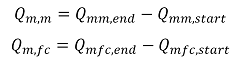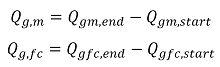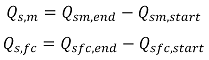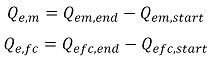# Metering Totals

## Mass Period TotalsSymbol Description Units
Qm,m Meter mass total kg
Qmm,end Meter mass total at end of period kg
Qmm,start Meter mass total at start of period kg
Qm,fc Flow Computer mass total kg
Qmfc,end Flow Computer mass total at end of period kg
Qmfc,start Flow Computer mass total at start of period kg

## Gross Volume Period TotalsSymbol Description Units
Qg,m Meter gross volume total kg
Qgm,end Meter gross volume total at end of period kg
Qgm,start Meter gross volume total at start of period kg
Qg,fc Flow Computer gross volume total kg
Qgfc,end Flow Computer gross volume total at end of period kg
Qgfc,start Flow Computer gross volume total at start of period kg

## Standard Volume Period TotalsSymbol Description Units
Qs,m Meter standard volume total kg
Qsm,end Meter standard volume total at end of period kg
Qsm,start Meter standard volume total at start of period kg
Qs,fc Flow Computer standard volume total kg
Qsfc,end Flow Computer standard volume total at end of period kg
Qsfc,start Flow Computer standard volume total at start of period kg

## Energy Period TotalsSymbol Description Units
Qe,m Meter energy total kg
Qem,end Meter energy total at end of period kg
Qem,start Meter energy total at start of period kg
Qe,fc Flow Computer energy total kg
Qefc,end Flow Computer energy total at end of period kg
Qefc,start Flow Computer energy total at start of period kg